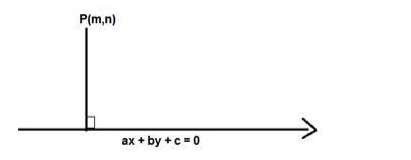# Find the distance of the point`
Question:

Find the distance of the point $(2,3)$ from the line $y=4$.

Solution:

Given: Point (2,3) and line y = 4

To find: The distance of the point (2, 3) from the line y = 4

Formula used: We know that the distance between a point $P(m, n)$ and a line $a x+b y+$ $c=0$ is given by,

$D=\frac{|a m+b n+c|}{\sqrt{a^{2}+b^{2}}}$The equation of the line is $y-4=0$

Here $m=2$ and $n=3, a=0, b=1, c=-4$

$D=\frac{|1(3)-4|}{\sqrt{0^{2}+1^{2}}}$

$D=\frac{|3-4|}{\sqrt{0+1}}=\frac{|-1|}{\sqrt{1}}=1$

$D=1$

The distance of the point (2, 3) from the line y = 4 is 1 units